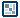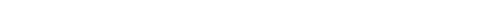## Question of the WeekRandom Numbers in Flash
written by kirupa, code by Scott Ruttencutter  |  10 January 2009

In programming, it is often nice to be able to work with numbers whose values are a bit unpredictable. To create numbers that occur with (almost) no set pattern, one would need to use a function that returns a random value.

Before I go further, here is a simple example where you will see a random number when you click on the button:

[ click on the Click Me button to see a random number between 0 and 100 ]

Generating a random number is pretty straightforward because a function for doing this already exists, and this function is:

Math.random();

In ActionScript, Math.random() returns a number greater than or equal to 0 but less than 1. Another way of stating that is (0 <=  n < 1) where n is the number you are looking for.

To configure a number to randomly fall within a range of numbers, use the following format:

Math.floor(Math.random()*(1+High-Low))+Low;

High refers to the largest number in your range, and Low refers to the lowest number in your range. For example, to get a number between 10 and 50, my line of code would look as follows:

Math.floor(Math.random() * 41) + 10;

A random number between 10 and 50 will be displayed. Scott goes into more detail in his blog post True Random Integers in Flash CS3, but I'll summarize this briefly.

Walking Through the Approach
You may be wondering why getting the a random number between a range of numbers is so...inelegant. The addition of the 1 seems almost silly in the grand scheme of things.

The reason is that Math.random() returns a number greater than or equal to zero AND less than 1. The function will never return a 1 as its value. It will return something like .9999999, but it won't be a 1. Because we are rounding the output by Math.floor where we round down to the nearest integer, if we did not add the 1, you will never get the maximum possible answer for High - Low when it is multipled by Math.random().

Let's say we ignore the one. Your code would now look as follows:

Math.floor(Math.random() * 40) + 10;

Math.random() * 40 will never return a 40 because that would require Math.random() to return a 1. We know from the AS3 documentation that Math.random() will get close to 1, but it will never reach one. Let's be optimistic and say that Math.random() returns a .9999, and we multiply that value by 40. The answer you will receive will be 39.996.

Guess what Math.floor of 39.996 is going to be? It is going to be 39! When this 39 gets added to 10, you get a value of 49. As you can see, you have no conceivable way of getting an answer of 50 which is the high number in your range of values. The only solution is to add a 1 to the operation where you are subtracting High from Low. Once you do that, you will be able to generate all numbers within the range of your high and low numbers with equal frequency.

Conclusion
Well, this wraps up my explanation of how random numbers can be generated in Flash. If you would like to see what my example looks like, download the source file from below:

Got a question or just want to chat? Comment below or drop by our forums (they are actually the same thing!) where a bunch of the friendliest people you'll ever run into will be happy to help you out!